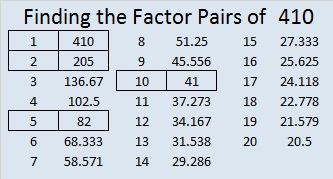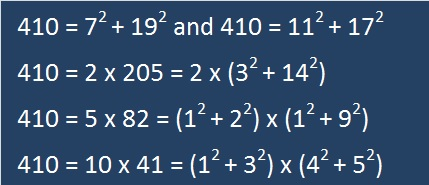# 410 Is The Sum of Two Squares Two Different Ways

410 = 59 + 61 + 67 + 71 + 73 + 79, six consecutive prime numbers.

410 = 199 + 211, two consecutive prime numbers.

• 410 is a composite number.
• Prime factorization: 410 = 2 x 5 x 41
• The exponents in the prime factorization are 1, 1, and 1. Adding one to each and multiplying we get (1 + 1)(1 + 1)(1 + 1) = 2 x 2 x 2 = 8. Therefore 410 has exactly 8 factors.
• Factors of 410: 1, 2, 5, 10, 41, 82, 205, 410
• Factor pairs: 410 = 1 x 410, 2 x 205, 5 x 82, or 10 x 41
• 410 has no square factors that allow its square root to be simplified. √410 ≈ 20.2485Those factors hardly seem like anything to write home about, but wait….How do I know all this? I applied something I read on Dr. David Mitchell’s blog, Lattice Labyrinths. He recently wrote a fascinating post about Tessellations and Pythagorean Triples. You have to read the article or at least look at his tessellations!

I didn’t know multiplying tessellating lattice labyrinths had anything to do with Pythagorean triples, but they do! (Actually I didn’t know anything about tessellating lattice labyrinths until I read the post.)

About the fourth or fifth paragraph of Dr. Mitchell’s post, he states something I didn’t know before: If you take one number that is the sum of two squares and find another number that is also the sum of two squares, and then if you multiply those two numbers together, that product will also be the sums of two squares! Since I knew both 10 and 41 could be written as the sum of two squares, I had to see if 410 could be also. I was doubly surprised and certainly not the least bit disappointed. I don’t know if it’s unusual that most of 410’s factors can also be written as the sum of two squares.

Because 410 and so many of its factors have that property, it is the hypotenuse of four non-primitive Pythagorean triples:

• [266-312-410] is [133 – 156 – 205] times 2.
• [246-328-410] is [3-4-5] times 82.
• [168-374-410] is [84-187-205] times 2.
• [90-400-410] is [9-40-41] times 10.

It is also the short leg of four other triples:

• [410-984-1066] is [5-12-13] times 82.
• [410-1656-1706] is [205-828-853] times 2.
• [410-8400-8410] is [41-840-841] times 10.
• [410-42024-42026] is [205-21012-21013] times 2.